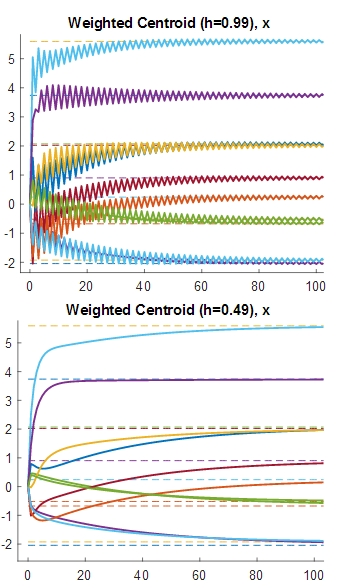# Paper: Distributed relative localization using the multi-dimensional weighted centroid

Title: Distributed relative localization using the multi-dimensional weighted centroid
Author: R. Aragüés, A. González, G. López-Nicolás, C. Sagüés.
Journal: IEEE Transactions on Control of Network Systems, vol. 7, pp. 1272-1282, 2020.Example with 10 agents in a chain graph. Evolution along iterations of the estimated x-coordinate relative to the weighted centroid of the team. Top: The ringing oscillatory behavior can be observed for h = 0.99. At each step, the estimates change their values sharply. Bottom: The ringing oscillatory behavior is removed with h = 0.49. The estimates converge nowsmoothly.

Abstract: A key problem in multi-agent systems is the distributed estimation of the localization of agents in a common reference from relative measurements. Estimations can be referred to an anchor node or, as we do here, referred to the weighted centroid of the multi-agent system. We propose a Jacobi Over–Relaxation method for distributed estimation of the weighted centroid of the multi-agent system from noisy relative measurements. Contrary to previous approaches, we consider relative multi-dimensional measurements with general covariance matrices not necessarily fully diagonal. We analyze the method convergence and provide mathematical constraints that ensure avoiding ringing phenomena. We also prove our weighted centroid method converges faster than anchor-based solutions.